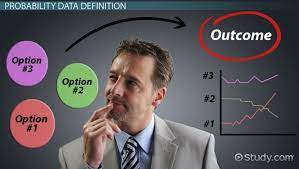Decision analysis allows corporations to evaluate and model the potential outcomes of various decisions to determine the correct course of action. To be effective, the business needs to understand multiple aspects of a problem to result in a well-informed decision.

The analysis entails understanding various goals, outcomes, and uncertainties involved, including the use of probabilities to measure the expected outcome of various decisions.

One of the most important aspects involves framing the problem in a way that allows for further analysis. Framing is typically the first part of decision analysis, and it involves creating a framework to evaluate the problem from multiple perspectives. They can include opportunity statements, action items, and measures of success.

Once the framework is established, a model can be developed to evaluate the favorability of various outcomes. Examples of models are decision trees and influence diagrams.

### Decision Trees

After creating a framework to evaluate a problem, models are typically used to evaluate the outcomes of various decisions. Models are visual representations of expected outcomes, and they are used to illustrate decisions in comparison to other alternatives.

By modeling the various expected outcomes and their probabilities, businesses can then select the decision that produces a favorable outcome.

One of the most common models involved in decision analysis is decision trees, which are tree-shaped models with “branches” that represent potential outcomes.

Decision trees are used because they are simple to understand and provide valuable insight into a problem by providing the outcomes, alternatives, and probabilities of various decisions. This makes it easy to evaluate which decision results in the most favorable outcome.

### Expected Value (EV)

After a model is constructed, it is important to find the expected value (EV) to evaluate which decision results in the most favorable outcome.

Recall that the decision trees provide all the possible outcomes in comparison to the alternatives. By calculating the expected value, we can observe the average outcomes of all decisions and then make an informed decision.

To calculate the expected value, we require the probability of each outcome and the resulting value. The formula for the expected value is as follows:

##### EV = (Probability A * Expected Payoff A) + (Probability B * Expected Payoff A)

The formula above assumes that a business decision has two outcomes – success or failure. Each outcome can be represented by Probability A or B. The Expected Payoff refers to the gain or loss expected with each outcome.

If there are multiple decisions to be made, a business will calculate the expected value for each decision to determine which is most favorable.

### Real-World Example

Let’s assume that a clothing store is opening a second location and wants to decide whether to open in San Francisco or New York. Opening a location in either city will involve different capital expenditures and demonstrate different rates of success.

Before constructing a decision tree, we will need to gather relevant data:

After gathering data, we can construct the decision tree based on each decision:

For each decision, the decision tree also includes numerical data to calculate the expected value. Squares represent decisions, and circles represent outcomes. The lines branching from squares are possible choices, while the lines branching from circles are expected outcomes.

The model also includes the costs associated with opening each location. To open in San Francisco, the store will need to invest \$2 million, while a New York location will require an investment of \$5 million.

The expected payoff amounts represent the potential revenue if the store succeeds, or the potential loss if the store fails.

To evaluate which decision is more favorable, we will calculate the expected value for each decision.

##### EV = (Probability A * Expected Payoff A) + (Probability B * Expected Payoff A)
• EV (San Francisco) = (0.4 * \$15,000,000) + (0.6 * -\$4,000,000) = \$3,600,000
• EV (New York) = (0.3 * \$30,000,000) + (0.7 * -\$10,000,000) = \$2,000,000

Then, we must deduct the initial capital expenditure to find the net gain/loss:

• San Francisco: \$3,600,000 – \$2,000,000 = \$1,600,000
• New York: \$2,000,000 – \$5,000,000 = -\$3,000,000## Next Generation Fixed Wireless Access (NGFWA) for Service Providers: A Viable Alternative to Traditional Fiber-Optic Networks

in## Transforming Nigeria’s Local Government System for Rural Economic Growth and Increased GDP

in